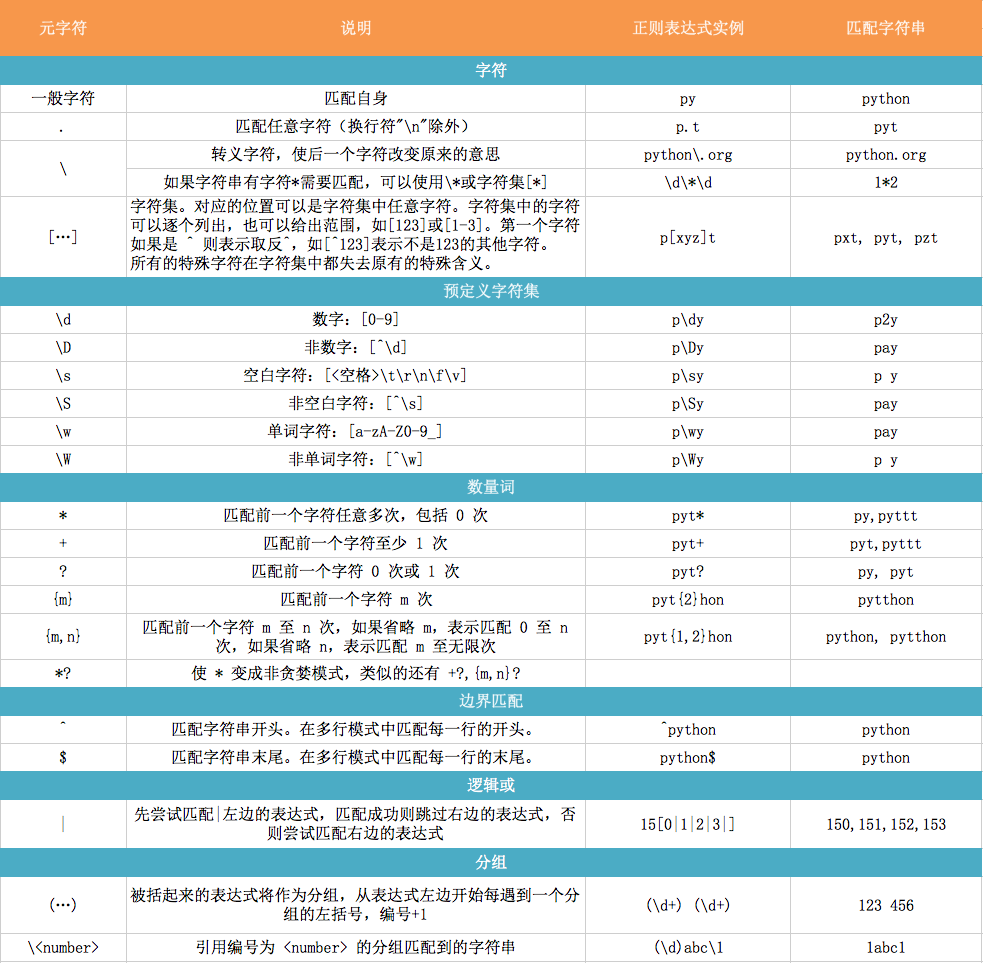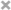### 参考链接

• http://funhacks.net/2016/12/27/regular_expression/
• http://www.runoob.com/python/python-reg-expressions.html

### pattern部分

flags的部分列举

• re.I 忽略大小写
• re.L 表示特殊字符集 \w, \W, \b, \B, \s, \S 依赖于当前环境
• re.M 多行模式
• re.S 即为 . 并且包括换行符在内的任意字符（. 不包括换行符）
• re.U 表示特殊字符集 \w, \W, \b, \B, \d, \D, \s, \S 依赖于 Unicode 字符属性数据库
• re.X 为了增加可读性，忽略空格和 # 后面的注释• \b，\B是单词边界，不匹配任何实际字符，所以是看不到的；\B是\b的非(补)。
• \b：表示字母数字与非字母数字的边界， 非字母数字与字母数字的边界。
• \B：表示字母数字与(非非)字母数字的边界，非字母数字与非字母数字的边界。

• \d{3}表示匹配3个数字，例如’010’；
• \s可以匹配一个空格（也包括Tab等空白符），所以\s+表示至少有一个空格，例如匹配’ ‘，’ ‘等；
• \d{3,8}表示3-8个数字，例如’1234567’

``````[0-9a-zA-Z\_]可以匹配一个数字、字母或者下划线；
[0-9a-zA-Z\_]+可以匹配至少由一个数字、字母或者下划线组成的字符串，比如'a100'，'0_Z'，'Py3000'等等；
[a-zA-Z\_][0-9a-zA-Z\_]*可以匹配由字母或下划线开头，后接任意个由一个数字、字母或者下划线组成的字符串，也就是Python合法的变量；
[a-zA-Z\_][0-9a-zA-Z\_]{0, 19}更精确地限制了变量的长度是1-20个字符（前面1个字符+后面最多19个字符）。
``````

A|B可以匹配A或B，所以(P|p)ython可以匹配’Python’或者’python’。

`````` ^表示行的开头，^\d表示必须以数字开头。
\$表示行的结束，\d\$表示必须以数字结束。
``````

**注意：使用Python的r前缀，就不用考虑转义的问题了，否则 `\b` 就要写成 `\\b` **

### re模块的分组——-group

``````>>> m = re.match(r'^(\d{3})-(\d{3,8})\$', '010-12345')
>>> m
<_sre.SRE_Match object at 0x1026fb3e8>
>>> m.group(0)
'010-12345'
>>> m.group(1)
'010'
>>> m.group(2)
'12345'
``````

``````>>> t = '19:05:30'
>>> m = re.match(r'^(0[0-9]|1[0-9]|2[0-3]|[0-9])\:(0[0-9]|1[0-9]|2[0-9]|3[0-9]|4[0-9]|5[0-9]|[0-9])\:(0[0-9]|1[0-9]|2[0-9]|3[0-9]|4[0-9]|5[0-9]|[0-9])\$', t)
>>> m.groups()
('19', '05', '30')
``````

### 贪婪匹配

``````>>> re.match(r'^(\d+)(0*)\$', '102300').groups()
('102300', '')
``````

``````>>> re.match(r'^(\d+?)(0*)\$', '102300').groups()
('1023', '00')
``````

### re 模块提供的函数

``````re 模块的使用步骤：
使用 compile 函数将正则表达式的字符串形式编译为一个 Pattern 对象
通过 Pattern 对象提供的一系列方法对文本进行匹配查找，获得匹配结果（一个 Match 对象）
最后使用 Match 对象提供的属性和方法获得信息，根据需要进行其他的操作
``````

### re.compile

compile 函数用于编译正则表达式，生成一个 Pattern 对象，供 match() 和 search() 这两个函数使用，它的一般使用形式如下：

``````re.compile(pattern[, flag])
``````

``````eg:
pattern = re.compile(r'\d+')
``````

``````match 方法
search 方法
findall 方法
finditer 方法
split 方法
sub 方法
subn 方法
``````

### match 方法

match 方法用于查找字符串的头部（也可以指定起始位置），它是一次匹配，只要找到了一个匹配的结果就返回，而不是查找所有匹配的结果。它的一般使用形式如下：

``````match(string[, pos[, endpos]])
``````

``````>>>import re
>>> pattern = re.compile(r'\d+')                    # 用于匹配至少一个数字
>>> m = pattern.match('one12twothree34four')        # 查找头部，没有匹配
>>> print m
None
>>> m = pattern.match('one12twothree34four', 2, 10) # 从'e'的位置开始匹配，没有匹配
>>> print m
None
>>> m = pattern.match('one12twothree34four', 3, 10) # 从'1'的位置开始匹配，正好匹配
>>> print m                                         # 返回一个 Match 对象
<_sre.SRE_Match object at 0x10a42aac0>
>>> m.group(0)   # 可省略 0
'12'
>>> m.start(0)   # 可省略 0
3
>>> m.end(0)     # 可省略 0
5
>>> m.span(0)    # 可省略 0
(3, 5)
``````

• group([group1, …]) 方法用于获得一个或多个分组匹配的字符串，当要获得整个匹配的子串时，可直接使用 group() 或 group(0)；
• start([group]) 方法用于获取分组匹配的子串在整个字符串中的起始位置（子串第一个字符的索引），参数默认值为 0；
• end([group]) 方法用于获取分组匹配的子串在整个字符串中的结束位置（子串最后一个字符的索引+1），参数默认值为 0；
• span([group]) 方法返回 (start(group), end(group))。

### search 方法

search 方法用于查找字符串的任何位置，它也是一次匹配，只要找到了一个匹配的结果就返回，而不是查找所有匹配的结果，它的一般使用形式如下：

``````search(string[, pos[, endpos]])
``````

``````>>> import re
>>> pattern = re.compile('\d+')
>>> m = pattern.search('one12twothree34four')  # 这里如果使用 match 方法则不匹配
>>> m
<_sre.SRE_Match object at 0x10cc03ac0>
>>> m.group()
'12'
>>> m = pattern.search('one12twothree34four', 10, 30)  # 指定字符串区间
>>> m
<_sre.SRE_Match object at 0x10cc03b28>
>>> m.group()
'34'
>>> m.span()
(13, 15)
``````

### findall 方法

``````findall(string[, pos[, endpos]])
``````

``````import re

pattern = re.compile(r'\d+')   # 查找数字
result1 = pattern.findall('hello 123456 789')
result2 = pattern.findall('one1two2three3four4', 0, 10)

print result1  # ['123456', '789']
print result2  # ['1', '2']
``````

### finditer 方法

finditer 方法的行为跟 findall 的行为类似，也是搜索整个字符串，获得所有匹配的结果。但它返回一个顺序访问每一个匹配结果（Match 对象）的迭代器。

``````re.finditer(pattern, string, flags=0)
``````

``````# -*- coding: utf-8 -*-
import re

pattern = re.compile(r'\d+')
result_iter1 = pattern.finditer('hello 123456 789')
result_iter2 = pattern.finditer('one1two2three3four4', 0, 10)
print type(result_iter1)
print type(result_iter2)
print 'result1...'
for m1 in result_iter1:   # m1 是 Match 对象
print 'matching string: {}, position: {}'.format(m1.group(), m1.span())
print 'result2...'
for m2 in result_iter2:
print 'matching string: {}, position: {}'.format(m2.group(), m2.span())
``````

``````<type 'callable-iterator'>
<type 'callable-iterator'>
result1...
matching string: 123456, position: (6, 12)
matching string: 789, position: (13, 16)
result2...
matching string: 1, position: (3, 4)
matching string: 2, position: (7, 8)
``````

### split 方法

split 方法按照能够匹配的子串将字符串分割后返回列表，它的使用形式如下：

``````split(string[, maxsplit])
``````

``````p = re.compile(r'[\s\,\;]+')
print p.split('a,b;; c   d')
#['a', 'b', 'c', 'd']
``````

### sub 方法

sub 方法用于替换。它的使用形式如下：

``````sub(repl, string[, count])
``````

``````import re

p = re.compile(r'(\w+) (\w+)')
s = 'hello 123, hello 456'
def func(m):
return 'hi' + ' ' + m.group(2)
print p.sub(r'hello world', s)  # 使用 'hello world' 替换 'hello 123' 和 'hello 456'
print p.sub(r'\2 \1', s)        # 引用分组
print p.sub(func, s)
print p.sub(func, s, 1)         # 最多替换一次
``````

``````hello world, hello world
123 hello, 456 hello
hi 123, hi 456
hi 123, hello 456
``````

### subn 方法

subn 方法跟 sub 方法的行为类似，也用于替换。它的使用形式如下：

``````subn(repl, string[, count])

(sub(repl, string[, count]), 替换次数)

``````

``````import re

p = re.compile(r'(\w+) (\w+)')
s = 'hello 123, hello 456'
def func(m):
return 'hi' + ' ' + m.group(2)
print p.subn(r'hello world', s)
print p.subn(r'\2 \1', s)
print p.subn(func, s)
print p.subn(func, s, 1)
``````

``````('hello world, hello world', 2)
('123 hello, 456 hello', 2)
('hi 123, hi 456', 2)
('hi 123, hello 456', 1)
``````

### re.match

• re.match 尝试从字符串的起始位置匹配一个模式，如果不是起始位置匹配成功的话，match()就返回none。
• 函数语法 ： re.match(pattern, string, flags=0)
``````import re
print(re.match('www', 'www.runoob.com').span())  # 在起始位置匹配          #(0, 3)
print(re.match('com', 'www.runoob.com'))         # 不在起始位置匹配        #None
``````

### re.search

re.search 扫描整个字符串并返回第一个成功的匹配。 `re.search(pattern, string, flags=0)`

``````>>> line = "Cats are smarter than dogs"
>>> matchObj = re.search( r'dogs', line, re.M|re.I)
>>> print "matchObj.group() : ", matchObj.group()
matchObj.group() :  dogs
``````
• re.match与re.search的区别: re.match只匹配字符串的开始，如果字符串开始不符合正则表达式，则匹配失败，函数返回None；而re.search匹配整个字符串，直到找到一个匹配。
• search 函数不能指定字符串的搜索区间，用法跟 Pattern 对象的 search 方法类似。

### re.findall

re.findall(pattern, string[, flags])

``````print re.findall(r'\d+', 'hello 12345 789')   #['12345', '789']
``````

### re.finditer

finditer 函数的使用方法跟 Pattern 的 finditer 方法类似，形式如下：

``````re.finditer(pattern, string[, flags])
``````

### re.split

``````re.split(pattern, string[, maxsplit])
``````

### re.sub

``````re.sub(pattern, repl, string[, count])
``````

### re.subn

``````re.subn(pattern, repl, string[, count])
``````

### 匹配中文

``````title = u'你好，hello，世界'
pattern = re.compile(ur'[\u4e00-\u9fa5]+')
result = pattern.findall(title)
print result
#  [u'\u4f60\u597d', u'\u4e16\u754c']
``````